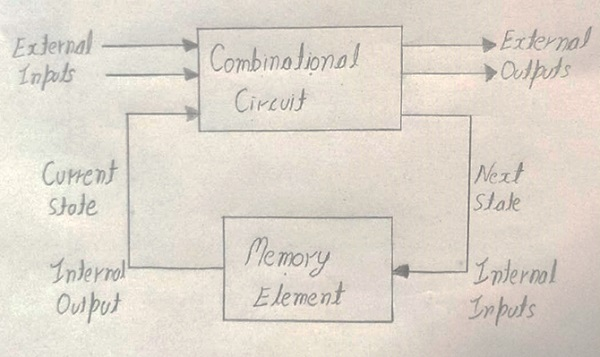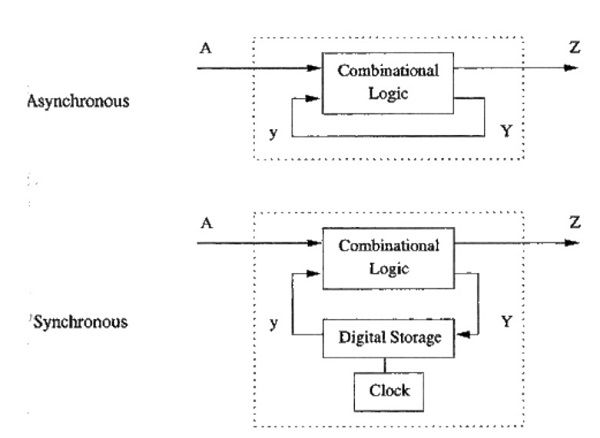# Sequential Circuits in Digital Electronics

Digital Electronics | Sequential Circuits: In this tutorial, we are going to read about the concept of Sequential Circuits, its types which are Asynchronous sequential circuits and Synchronous sequential circuit. Also, we will discuss the differences between Combinational circuits and Sequential circuits.
Submitted by Saurabh Gupta

## Introduction to Sequential Circuits

The word sequential circuit means "a circuit whose output depends on the order or timing of the inputs".

So, the sequential circuit consists of Y number of inputs, logic gates, and X number of outputs.

Sequential circuits produce output which depends upon current inputs and previous input variables. Thus, we can say that sequential circuits are capable of storing binary information. This binary information gives the state of the sequential circuit at that time. The block diagram of sequential circuits is given below,From the above figure, we can see that there are two types of input present. They are:

1. External inputs which are not controlled by the circuit and do not depend on past input.
2. Internal inputs depend on past inputs.

## Types of Sequential Circuits

There are two types of sequential circuits which we study in digital electronics which are listed below,

1. Asynchronous sequential circuit
2. Synchronous sequential circuit

### 1) Asynchronous sequential circuit

The asynchronous sequential circuit does not use a clock signal but they use the pulse of inputs. If we compare between Asynchronous sequential circuit and Synchronous sequential circuit, the Asynchronous sequential circuit has a clock pulse and it is capable of changing state immediately when there is a change in the input signal. Asynchronous sequential circuits are used where the speed of operation is important. It has a disadvantage that designing this circuit is difficult and output is uncertain.

### 2) Synchronous sequential circuit

The synchronous sequential circuit uses clock signal and level inputs or pulsed inputs (which has a restriction on pulse width and circuit propagation). The output pulse takes the same duration as the clock pulse for the clocked sequential circuits. If we compare Synchronous sequential circuit and Asynchronous sequential circuit than Asynchronous sequential circuit is faster because in Synchronous sequential circuit they have to wait for the next clock pulse to arrive to perform the next operation, so Synchronous sequential circuit becomes a little bit slower. In this level of output change state happen at the starting of the input pulse and remain the same until the next clock pulse or input pulse come.

They are used in flip flops and to design counters, registers, RAM, MOORE machines, and other state retaining machines.

The block diagram of Asynchronous and Synchronous sequential circuits## Difference between Combinational Circuits and Sequential Circuit

SrNo Combinational Circuits Sequential Circuits
1 Output depends upon present input Output depends upon present and past input
2 Working is fast Working is slow
3 Easy to design Complex to design
4 Time independent Time-dependent
5 Used in arithmetic operation Used in storing data
6 Don’t have a clock Have a clock
7 Easy to use and handle Difficult to use and handle
8 Example: Encoder, Decoder, Multiplexer Example: Flip-Flop, Counters

### Application of Sequential Circuits

Sequential circuits are used at several places some of which are listed below:

• They are applied in programmable devices, for example, CPLD (Complex Programmable Logic Device), PLD (Programmable Logic Device), and FPGA (Field Programmable Gate-Array).
• They are used as registers in microprocessors.
• They are also used as controllers for storing temporary information.
• Shift registers, Flip – Flops, Clocks, Counters.
• Conversion of analog signals to digital signals and vice-versa.